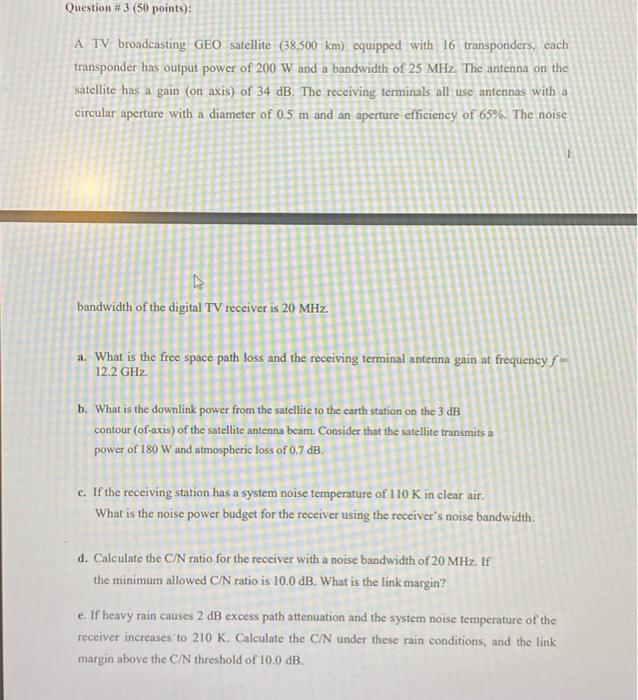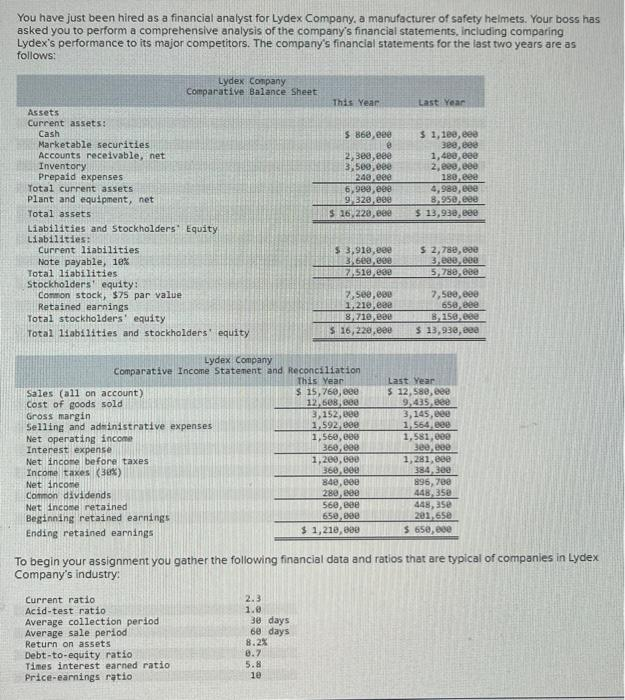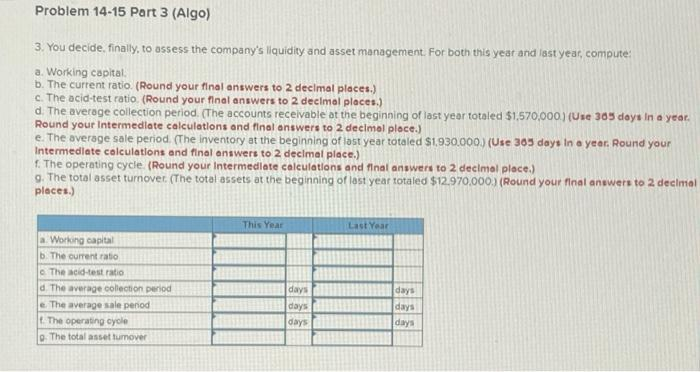### (Solved): Question \#3 (50 points): A TV broadcasting GEO satellite (...Question \#3 (50 points): A TV broadcasting GEO satellite equipped with 16 transponders, each transponder has output power of and a bandwidth of . The antenna on the satellite has a gain (on axis) of . The receiving terminals all use antennas with a circular aperture with a diameter of and an aperture efficiency of . The noise i bandwidth of the digital TV receiver is a. What is the free space path loss and the receiving terminal antenna gain at frequency . b. What is the downlink power from the satellite to the earth station on the contour (of-axis) of the satellite antenna beam. Consider that the satellite trinsmits a power of and atmospheric loss of . c. If the receiving station has a system noise temperature of in clear air. What is the noise power budget for the receiver using the receiver's noise bandwidth. d. Calculate the ratio for the receiver with a noise bandwidth of . If the minimum allowed ratio is . What is the link margin? e. If heavy rain causes excess path attenuation and the system noise temperature of the receiver increases to . Calculate the under these rain conditions, and the link margin above the threshold of .

### (Solved): project: Design and build(simulate) a 4-bit counter: --it mu...

project: Design and build(simulate) a 4-bit counter: --it must not be build using JK-flip-flop (any other type) --it must flip on 3,4,13

### (Solved): Calculate the voltage generated by a fuel cell at 85 A/m^2...

Calculate the voltage generated by a fuel cell at 85 A/m^2 using the equations given . the following assumptions are made to simplify the analysis of PEM fuel cell stack . (1) The system reaches a steady state. (2) Air in the fuel cell consists of 79% N2 and 21% O2. (3) Chemical reaction reaches equilibrium state. (4) Constant and equal pressures in the fuel cell gas flow channels. (5) The fuel is humidified hydrogen and oxidant is humidified air. Assume the effective anode water vapor pressure is 50% of the saturated vapor pressure while the effective cathode water pressure is 100%. (6) The fuel cell operating temperature keeps at 85 C, and fuel cell operating pressure is 303.96 kPa. (7) Flow temperature at the exit of the channels is constant and equal to the operating temperature of stack. (8) The temperature is distributed uniformly across the stack and the overall specific heat capacity is assumed to be a constant. (9) Pressure change at PEM fuel cell stack is negligible. (10) The heat loss to surroundings is neglected due to the insulation layer. The organic working fluids are chosen to instead of the conventional coolant for the PEM fuel cell stack heat dissipation. (11) Parameters for single cell can be lumped together to form a stack. (12) The mechanical conversion efficiency of compressor is assumed to be 100%.

### (Solved): help plz You have just been hired as a financial analyst fo...

help plz